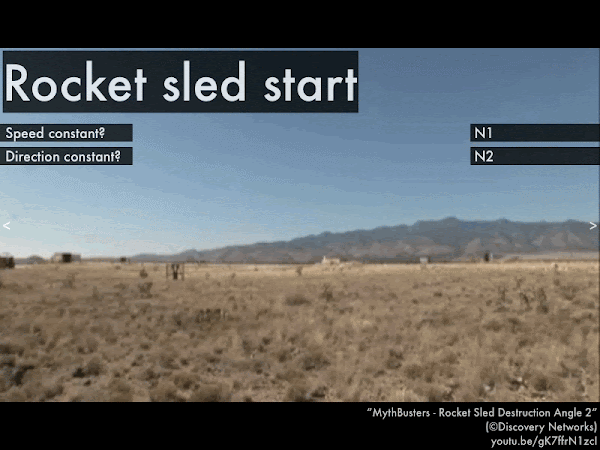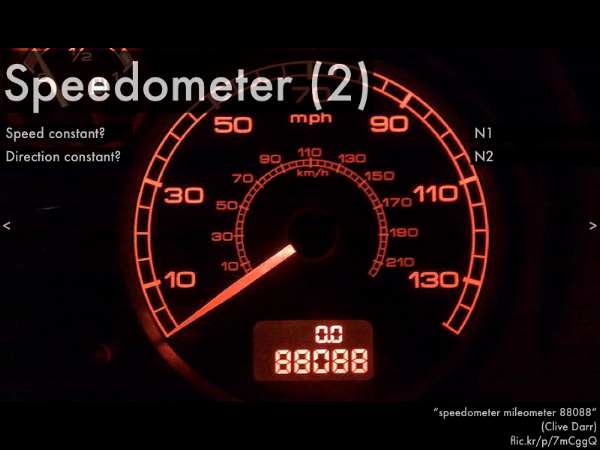## 20170918

### Online reading assignment: forces and motion

Physics 205A, fall semester 2017
Cuesta College, San Luis Obispo, CA

Students have a bi-weekly online reading assignment (hosted by SurveyMonkey.com), where they answer questions based on reading their textbook, material covered in previous lectures, opinion questions, and/or asking (anonymous) questions or making (anonymous) comments. Full credit is given for completing the online reading assignment before next week's lecture, regardless if whether their answers are correct/incorrect. Selected results/questions/comments are addressed by the instructor at the start of the following lecture.

The following questions were asked on reading textbook chapters and previewing a presentation on forces and motion.Selected/edited responses are given below.

Describe what you understand from the assigned textbook reading or presentation preview. Your description (2-3 sentences) should specifically demonstrate your level of understanding.
"I understand that if the net forces add up to zero we use newtons first law and the acceleration for the object has to equal zero."

"Newton's laws pertain to motion primarily; the first law pertaining to a zero net force and the second law being a non-zero net force case."

"If the speed is constant AND the direction of motion is constant, then it is Newton's first law. If either of those are a no then Newton's second law applies."

"The net force equals the sum of all the forces acting on an object."

"The motion flow chart helped me to understand Newton's laws a little bit. Or at least be able to tell them apart."

Describe what you found confusing from the assigned textbook reading or presentation preview. Your description (2-3 sentences) should specifically identify the concept(s) that you do not understand.
"Can you do more stuff on the angles. The angles are very confusing for me."

"Newton's second law of motion, because I don't understand the relation between the mass, acceleration and the SI unit for force or net force."

"I spent a lot of time on the reading, as well as I learned this stuff in high school, but I am having trouble understanding the overall concept and application of net force and motion."

"Not entirely sure the difference between Newton's first and second laws. I think the third law throws me off more too."

"I'm a little confused on what net force is and how to apply it."

"To be honest the textbook is a bit confusing, more lecture is needed."

"I need more help finding hints to if I'm looking at a first law or a second law situation."

"Don't really understand the second law of motion."

"I am confused by the connection between net force and Newton's first and second laws."

"I didn't find too much of the information confusing, I would just benefit from more clarification on Newton's first and second laws."

"Everything was clear."

Identify whether a zero or non-zero net force corresponds to Newton's first law or Newton's second law. (Only correct responses shown.)
Zero net force (ΣF = 0): Newton's first law ("N1") [91%]
Non-zero net force (ΣF ≠ 0): Newton's second law ("N2") [91%]For the rocket sled, Newton's __________ law applies to the motion of this object, and the forces acting on the object add up to a __________ net force.
 first; zero. *************  second; non-zero. *************************  (Unsure/guessing/lost/help!) **While the F-35B is descending (before it touches the ground), Newton's __________ law applies to the motion of this object, and the forces acting on the object add up to a __________ net force.
 first; zero. ********************  second; non-zero. ***********************  (Unsure/guessing/lost/help!) **For this car with a steady speedometer reading (and assuming the shaking is due to the person holding camera, and not from moving the car itself), Newton's __________ law applies to the motion of this object, and the forces acting on the object add up to a __________ net force.
 first; zero. ***********************************  second; non-zero. ********  (Unsure/guessing/lost/help!) **For a person in the swinging chair ride moving along a circular trajectory at a constant speed, Newton's __________ law applies to the motion of this object, and the forces acting on the object add up to a __________ net force.
 first; zero. *************  second; non-zero. ******************************  (Unsure/guessing/lost/help!) **For this car with a zero speedometer reading, Newton's __________ law applies to the motion of this object, and the forces acting on the object add up to a __________ net force.
 first; zero. ***********************************  second; non-zero. *****  (Unsure/guessing/lost/help!) ***** 

Ask the instructor an anonymous question, or make a comment. Selected questions/comments may be discussed in class.
"So anytime an object is moving at a constant velocity is it experiencing Newton's first law? And anytime an object is accelerating it is experiencing Newton's second law?" (Yes, and yes.)

"For the rocket sled start, it is accelerating right? Therefore it should be Newton's second law? I have a lot of hesitation about this one." (Yes. Relating motion with Newton's first and second laws seems like it shouldn't be a big deal, but it's a important step we'll need to concentrate on before we start connecting it with net forces and free body diagrams.)

"If the speed is zero...it's still a constant speed of zero, right?" (Yes.)

"Unless it says constant velocity or at rest, it's Newtons second law right?" (Yes.)

"I actually found the question about the spinning swing a little confusing. The speed is constant and it is constantly moving in a circle; so does that mean that the direction is constant or because it is a circle the direction does change?" (The direction of velocity is always changing, as at every moment in time, this (straight-line) vector points in a different direction, tangent to the curve of the circle.)

"The more real-life examples you give us there better I learn and remember."

"Can you lecture more on this?"

"I get what's going on from a calculus standpoint and a physical standpoint separately. Melding the two into a picture and applying the necessary equations isn't incredibly challenging, but takes a lot more time than I expect is normal for these types of problems. I could benefit greatly just from more question sets online or in class."

"What surface would be close enough to frictionless so that you could move a cart with low mass and have it keep going for a super-long distance without exerting much force?" (An amazing new material called SLIPS, appropriately enough. Because it's so slippery, it stays very clean, as virtually nothing will stick to it. On a macabre note, it works just like the sides of a carnivorous pitcher plant thats trap insects, so insects can't stick to its sides and crawl out.)

"Even though the flipped class is not the easiest way for me to learn, the organisation of the lab makes me learn a lot more than if it was lectured."

"What is the silver-and-black thing that you wear on your collar?" (It's a microphone for my CROS (contralateral routing of signal) hearing aid; I have idiopathic single-sided sensorineural hearing loss.)